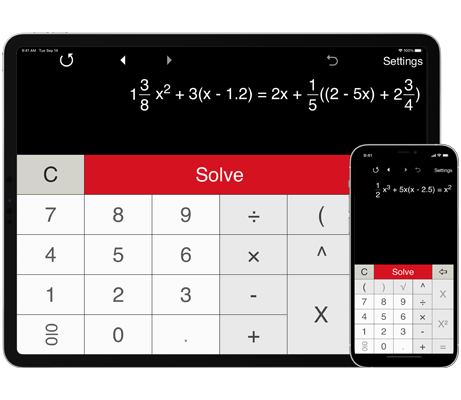# Solving linear equations with fractions

Linear equation calculator with steps helps you solve linear equations in one variable, including linear equations with fractions.

## Solving linear equations with one variable containing fractions

Linear equations calculator can be used to solve linear equations or first-degree equations with one variable containing fractional coefficients, including mixed fractions.

Pre-defined formats for solving linear equations with fractions in the form ax + b = c and ax + b = cx + d. For any coefficient you can enter a whole number, a mixed number, or a fraction. Coefficients can be negative.

With the Advanced format that could be changed in the Settings window, you can input your equation in any form.